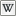# LSL Operators

 LSL Portal

Operators are used to cause an operation (or mathematical action) to be performed on one (such as !) or two operands. The easy and common example is 1 + 2 where 1 and 2 are operands, and the + is the operator. This concept can be extended much further with LSL since operands can be variables with the special case of the assignment operators requiring that the left hand side be a variable.

The following table lists the operators in descending order of evaluation, i.e. higher in the table means higher evaluation precedence. Multiple operators on the same line share evaluation precedence. Parenthesize an expression if you need to force an evaluation order.

Operator Description Usage Example
`()` parentheses: grouping and evaluation precedence `integer val = a * (b + c);`
`[]` brackets: list constructor `list lst = [a, 2, "this", 0.01];`
`(type)` typecasting `string message = "The result is:" + (string)result;`
`!` `~` `++` `--` logical NOT, bitwise NOT, increment, decrement `counter++;`
`*` `/` `%` multiply/dot product, divide, modulus/cross product `integer rollover = (count + 1) % 5;`
`-` subtraction, negation `integer one = 3 - 2;`

`integer neg_one = -1;`

`+` addition, string concatenation `integer two = 1 + 1;`

`string text = "Hello" + " world";`

`+` list concatenation `list myList = [1, 2, 3] + [4, 5];`

`list newList = oldList + addList;`

`<<` `>>` arithmetic left shift, arithmetic right shift `integer eight = 4 << 1;`

`integer neg_one = -2 >> 1;`

`<` `<=` `>` `>=` less than, less than or equal to, greater than, greater than or equal to `integer isFalse = (6 <= 4);`
`==` `!=` comparison: equal, not equal `integer isFalse = ("this" == "that");`
`&` bitwise AND `integer zero = 4 & 2;`

`integer four = 4 & 4;`

`^` bitwise XOR `integer zero = 4 ^ 4;`

`integer six = 4 ^ 2;`

`|` bitwise OR `integer four = 4 | 4;`

`integer six = 4 | 2;`

`||` logical OR `integer isTrue = (FALSE || TRUE);`
`&&` logical AND `integer isFalse = (FALSE && TRUE);`
`=` `+=` `-=` `*=` `/=` `%=` assignment `integer four = 4;`

`integer eight = four; eight *= 2;`

Note: Modulus (`%`), like division, cause a Script run-time error. Math Error when its second operand equals zero.

Note: The `%` operator only accepts integer (`%` as modulus) and vector (`%` as cross product) operands.

Note: Unlike most other languages that use the C-style `&&` and `||` operators, both operands are always evaluated. For example,

```if (TRUE || 1/0) llSay(PUBLIC_CHANNEL, "Aha!");
```
will cause a Math Error rather than say "Aha!"

Note: The `++` (increment) and `--` (decrement) operators have two versions, pre- and post-. The pre-increment (or pre-decrement) operator increments (or decrements) its operand by 1; the value of the expression is the incremented (or decremented) value. The post-increment (or post-decrement) operator increases (or decreases) the value of its operand by 1, but the value of the expression is the operand's original value prior to the operation.

```integer count = 0;
if( ++count == 1 ) // 'count' is incremented then evaluated.
llSay(PUBLIC_CHANNEL, "Aha"); // message will be said.
```
```integer count = 0;
if( count++ == 1 ) // 'count' is evaluated then incremented.
llSay(PUBLIC_CHANNEL, "Aha"); // message will not be said.
```

Note: The order of precedence of boolean operators is unclear. It is possible that there is a bug in the expression parser, making precedence inconsistent, or it may simply be that || and && have equal precedence; testing is inconclusive. Thus, when in doubt, parenthesize. SubNote: As the above bug has been closed as expected behavior, one can only assume the boolean operators have equal precedence.

Note: The order of evaluation appears to be backwards from most languages. If the value of x starts as 1 then the first two conditions below evaluate false and the second two evaluate true:

```(x && (x = 0) == 0 && x)
```
```(x && (x = 0) == 0 && x == 0)
```
```(x == 0 && (x = 0) == 0)
```
```(x == 0 && (x = 0) == 0 && x)
```

Both sides are evaluated regardless of the the truth of either side.

Note: Equality test on lists does not compare contents, only the length.

## + Operator

`result = left + right`

Left Type Right Type Result Type Description
integer integer integer Adds left and right
integer float float Adds left and right
float integer float Adds left and right
string string string Concatenates right onto the end of left.
list * list Concatenates right onto the end of left.
* list list Affixes left onto the start of right.
vector vector vector Adds left and right
rotation rotation rotation Adds left and right
Not useful for combining rotations, use * or / instead.

## Shorthand Operators

Alternatives to the simple '=' operator...

Simple assignment operator Shorthand operator
a = a + 1 a += 1
a = a – 1 a -= 1
a = a * (n+1) a *= (n+1)
a = a / (n+1) a /= (n+1)
a = a % b a %= b

##De Morgan's laws

Bitwise Equivalencies
AND OR
`~(a & b)` `~a | ~b`
`~a & ~b` `~(a | b)`
`a & ~b` `~(~a | b)`
`~(a & ~b)` `~a | b`
Boolean Equivalencies
AND OR
`!(a && b)` `!a || !b`
`!a && !b` `!(a || b)`
`a && !b` `!(!a || b)`
`!(a && !b)` `!a || b`

Due toDe Morgan's laws, by row, code in the AND column is logically equivalent to code in the OR. a and b need not be variables, they can be expressions. In certain circumstances these equivalencies can be used to simplify complex code. It is important not to confuse the two sets when using them. The first two rows depict De Morgan's laws as it is formulated, the second two build upon it.